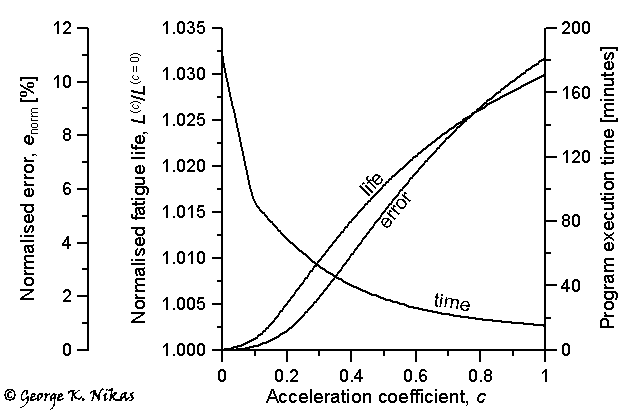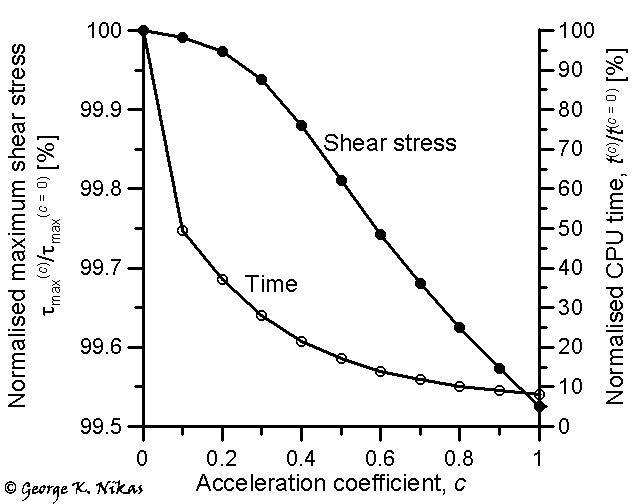Paper: Boussinesq-Cerruti functions and a simple technique for substantial acceleration of subsurface stress computations in elastic half-spaces.

Author: George K. Nikas

Abstract

The Boussinesq-Cerruti potential functions for the calculation of subsurface stresses and displacements in elastic half-spaces are presented in full and in clear formulation. They were expanded and optimised for fast computational analysis of subsurface stress and displacement fields, including fatigue life computations. A simple technique was developed to accelerate the computations by omitting the stress contribution of parts of the loaded boundary if the said contribution is lower than a pre-determined limit. The error of this approximation was defined and formulated for comparison of the various solutions. The technique was applied in a computationally intensive problem involving a rolling-sliding-spinning elastohydrodynamic, elliptical, heavily loaded contact, and three-dimensional subsurface stress and displacement fields were calculated with hundreds of thousands of surface gridpoints and tens of thousands of subsurface gridpoints, followed by computation of the fatigue lives of the contacting solids. Results are presented for a smooth contact but the method has been applied and is particularly useful for rough contacts as well. The results show a two to twenty fold acceleration of computations, which reduces the computational times by 50 to 95% for a negligible to small loss of accuracy. In real terms this means reduction of computer time from days to hours or from hours to minutes.

Some figures from this work

The computational technique was originally developed to deal with excessive CPU times when computing three-dimensional subsurface stress fields in elastohydrodynamic elliptical contacts, especially in rough contacts where the computational grids are dense to properly deal with roughness asperity effects. In such cases and when using hundreds of thousands of gridpoints to capture micro-scale effects, a dramatic reduction of CPU time is achieved by using the proposed technique. Figure 1 shows such an example. It refers to the elastohydrodynamic elliptical contact between a roller and a disc in the variator of an Infinitely Variable transmission (see details and stress fields from that project). The contact is assumed smooth and the kinematics involve simultaneous rolling, sliding and spinning. Subsurface stress fields for steady-state conditions were computed with 9,000 subsurface gridpoints for each of the two cooperating bodies and 152,100 gridpoints on the surface of each body.Fig. 1. Effect of the acceleration coefficient on predicted fatigue life (PC: 1.5 GHz Intel Pentium-4 processor).

The acceleration coefficient on the horizontal axis in Fig. 1 is a measure of how many surface gridpoints are ignored when calculating subsurface stresses, based on the selection criterion of the proposed technique. The greater the acceleration coefficient, the greater the number of surface gridpoints omitted. As shown in Fig. 1, this has a substantial effect on the program execution time. The error of this approximation is also formulated in the paper and shown in Fig. 1, together with the result of the predicted, normalised fatigue life of one of the solids (results are similar for the other solid), using the Ioannides-Harris fatigue life model. The normalised fatigue life, which is the computed fatigue life L(c) for acceleration coefficient c over the computed fatigue life L(c = 0) without accelerated computations (that is, for the full analysis), shows a negligible difference of up to about 3% in Fig. 1. The normalised error of the approximation (Fig. 1) is also very small, yet the reduction of CPU time, even for a small acceleration coefficient c = 0.2 is substantial.

The article contains figures showing the variation of all six components  of the stress tensor with the acceleration coefficient (including minimum, maximum and average values), where the negligible effect on accuracy and the substantial reduction of CPU time are clear to see. Apart from those, it is interesting from an engineering perspective to see the effect of the acceleration technique on the maximum shear stress (which is often used for fatigue life estimation or evaluation of the risk of damage via the Tresca criterion). This is shown in Fig. 2.Fig. 2.  Effect of the acceleration coefficient on the maximum shear stress (refer to Fig. 1).

As is realised from Fig. 2, even for a relatively high acceleration coefficient c = 1, the error in the computation of the maximum shear stress is lower than 0.5% and the corresponding reduction of computing time is 92%. For even greater (but rather unnecessary) precision, one could use c = 0.1 and achieve a 50% reduction of CPU time with only 0.01% of error in the computation of the maximum shear stress as shown in Fig. 2.

Applying the technique to the same problem as above but including surface roughness effects, with substantially increased numbers of gridpoints (nearly 1 million surface gridpoints and 36,000 subsurface gridpoints for each solid), the stress analysis takes 52 hours of CPU time with zero acceleration on a 1.5 GHz Pentium-4 processor, yet it takes only 2 hours and 49 minutes when using an acceleration coefficient c = 1.0, without unacceptable loss of accuracy. This is obviously a substantial improvement, without which the subsurface stress analysis is rather unfeasible (52 hours of CPU time equate to about 2.2 days; that is continuous, uninterrupted running time with 100% usage of an Intel Pentium-4 processor, running at 1.5 GHz for 2.2 days).

Homepage of Dr Nikas